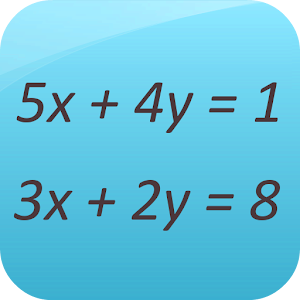## Linear Equation System Solver - Lumos Educational App Store3.71
Price -FREE
\$0

#### DESCRIPTION:

This app is a free math calculator which is able to solve systems of linear equations. You can solve: -Systems of two variables and two equations -Systems of three variables and two equations -Systems of three variables and three equations -Systems of four variables and two equations -Systems of four variables and three equations -Systems of four variables and four equations -Systems of four variables and four equations -Systems with n variables and m equations (more than 4) Best mathematical tool for school and college! If you are a student, it will help you to learn linear alge

#### OVERVIEW:

Linear Equation System Solver is a free educational mobile app By GK Apps .It helps students in grades HS practice the following standards A.CED.A.3.

This page not only allows students and teachers download Linear Equation System Solver but also find engaging Sample Questions, Videos, Pins, Worksheets, Books related to the following topics.

HS

#### STANDARDS:

A.CED.A.3

Developer: GK Apps

Software Version: 3.7

Category: Education

### RELATED APPSEdSearch WebSearch